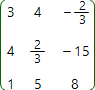# Characteristic polynomial online calculatorCharacteristic polynomial of the matrix A, can be calculated by using the formula:

| Aλ E |

where E - identity matrix, which has the same number of rows and columns as the initial matrix A.

Look closer at the formula above. If matrix A is of the form:then expression Aλ E has the form:Finally, we should find the determinant:After calculating the determinant, we'll get the polynomial of n-th degree (n - order of initial matrix), which depends on variable λ:

P( λ ) = cn λn + cn1 λn1 + ... + ci λi + ... + c1 λ + c0

As soon as to find characteristic polynomial, one need to calculate the determinant, characteristic polynomial can only be found for square matrix.

Our online calculator is able to find characteristic polynomial of the matrix, besides the numbers, fractions and parameters can be entered as elements of the matrix.

Characteristic polynomial calculator
- use Sarrus rule to calculate third order determinants.A =